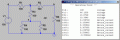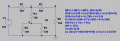# Thevenins Equivalent Circuit

## Thevenins Equivalent circuit

• ### Thevenin

• Total voters
0

#### JIMMYR10

Joined Jan 25, 2015
11
I need to find the Thevenins Equivalent for the above circuit.

I am pretty confident that I have worked out the Equivalent voltage but am unsure of the voltage

Any help would be much appreciated

Thanks

Moderators note: reduced picture size.

#### Attachments

Last edited by a moderator:

#### JIMMYR10

Joined Jan 25, 2015
11
I need to find the Thevenins Equivalent for the above circuit.

I am pretty confident that I have worked out the Equivalent voltage but am unsure of the voltage

Any help would be much appreciated

Thanks

Sorry I meant I have worked out the resistance

#### rithup

Joined Jan 25, 2015
2

#### ErnieM

Joined Apr 24, 2011
8,362
37.5 V and Ohms

@rithup: don't forget the 2nd equivalent also loads the first.

#### rithup

Joined Jan 25, 2015
2
I am confused now. Could you please elaborate?

37.5 V and Ohms

@rithup: don't forget the 2nd equivalent also loads the first.

#### JIMMYR10

Joined Jan 25, 2015
11
Thanks guys @ErnieM @rithup

It seems I have the resistance correct but I am not sure where I am going wrong on the voltage.

Moderators note: cropped and reduced size of image

#### Attachments

Last edited by a moderator:

#### ErnieM

Joined Apr 24, 2011
8,362
It is nearly impossible to describe a circuit that has no reference designations.

Add some and I will attempt.

#### JIMMYR10

Joined Jan 25, 2015
11
Thanks @ErnieM I really appreciate your help.

Hopefully this picture is better for you.

Please let me know if there is anything else you need.

I had a go earlier and I think I may have worked out how you got to 30v but am unsure about how you would get to 37v

Many thanks

Moderators note: reduced size of image

#### Attachments

Last edited by a moderator:

#### WBahn

Joined Mar 31, 2012
29,489
Do you notice that moderators are having to constantly resize your images? In the future, please be considerate of their time and size your images appropriately before attaching them. As you can see, it is entirely possible to get workable images in well under 100kB. You can use Paint or similar free programs to do this.

#### WBahn

Joined Mar 31, 2012
29,489
Shouldn't this be in Homework Help???

If you were not being asked to find a Thevenin equivalent, but instead just ask to take that circuit and fine the voltage across the far right 100Ω resistor, could you do it? How might you go about doing that?

#### JIMMYR10

Joined Jan 25, 2015
11
Sorry @WBahn I am new to the forum

Do I just add all of the resistors and divide by the voltage?

#### WBahn

Joined Mar 31, 2012
29,489
Sorry @WBahn I am new to the forum

Do I just add all of the resistors and divide by the voltage?
I don't even know what that could possibly mean. You are looking for a voltage and dividing any resistance by any voltage is not going to give you a voltage.

Usually before you get to Thevenin equivalent circuits you have been through analyzing DC circuit using several different techniques ranging from direct application of KVL and KCL, branch currents, loop voltages, Node Voltage Analysis, Mesh Current Analysis, and superposition. Do any of those ring a bell?

#### ke5nnt

Joined Mar 1, 2009
384
I put this together when I was in my DC Circuits class. Perhaps it will be of help to you.

#### Attachments

• 202.7 KB Views: 44

#### JIMMYR10

Joined Jan 25, 2015
11
I'll be honest with you the only one I've heard of is superposition.

I'm on a mechanical engineering course and there's just a small unit on Electrical science.

I've done Some Thevenin equivalent circuits but they were more simple than this.

I've looked everywhere for something similar but just can't seem to get there.

Like I say I've got the voltage and could see how someone could get to a voltage of 30v but I don't know if this is correct unless someone can help me

#### MikeML

Joined Oct 2, 2009
5,444
Here is the "correct" answer for the "no-load" Thev. Equiv. output voltage:You should be able to arrive at the same answer algebraically using only Ohm's & Kirchoff's Current Law, and simple combining of resistances.I'll let you find the Thev. Equiv. resistance.

Last edited:
•JIMMYR10

#### Glenn Holland

Joined Dec 26, 2014
703
The "Thevenin Equivalent" circuit contains only one voltage source connected to only one resistor in series. Using the Thevenin equivalent circuit simplifies calculations where the load resistance is a variable quantity that is connected to a "black box" source.

The process for converting a complex circuit to an equivalent circuit is as follows:
• Remove whatever is considered to be the "external resistive load" from the network. In your drawing, it looks like terminals A and B are where that load would be connected.
• Calculate the voltage of the network at terminals A and B. This will be the “Thevenin Voltage” source.
• Replace all the voltage sources with just a solid wire, then calculate the resistance of the network as measured at terminals A and B. This resistance will be the “Thevenin Resistance”.
• Connect the Thevenin voltage source and its resistance in series. You now have the Thevenin Equivalent Circuit.

Last edited:

#### WBahn

Joined Mar 31, 2012
29,489
I'll be honest with you the only one I've heard of is superposition.

I'm on a mechanical engineering course and there's just a small unit on Electrical science.

I've done Some Thevenin equivalent circuits but they were more simple than this.

I've looked everywhere for something similar but just can't seem to get there.

Like I say I've got the voltage and could see how someone could get to a voltage of 30v but I don't know if this is correct unless someone can help me
Okay, surely you've done Kirchhoff's voltage and current laws as part of Physics II (at least most curricula require that for mech. eng.). I'm assuming you're also familiar with Ohm's Law.

Let's call node B our "ground", so it has a voltage of 0V and we are looking for the voltage at node A.

Let's call the node at the top of the battery node C and the node at the tee between the top two resistors node D.

If I were to tell you what the voltage is at node D, could you find the voltage at node A?

If so, then our problem is reduced to finding the voltage at node D.

If I were to tell you the current flowing in the battery, could you find the voltage at node D?

If so, the our problem is reduced to finding the current flowing in the battery.

If I told you the equivalent resistance of the entire circuit as seen by the battery, could you find the current flowing in the battery?

If so, then our problem is reduced to finding the equivalent resistance of the circuit as seen by the battery.

Given the prior work you've shown, I think you have the knowledge and skills to do that. So work start from this last step and work your way back.

Do you see the basic problem solving approach at work here? Start with what you want to know and then ask yourself what one or two pieces of information would make solving the problem easy if you only knew those pieces of information. Treat that as a new problem as do the same thing. Eventually you get to something that requires only information you already have or that you have the knowledge and skills to answer readily.

Do the best you can, show your work, and we can go from there.

#### ErnieM

Joined Apr 24, 2011
8,362
Hopefully this picture is better for you.
That is just perfect.

There are many advanced ways to analize a complicated circuit that tend to be a very mechanical process. This circuit is a bit different because it can easily be split into pieces and each piece is a simple analysis.

Draw a mental line down between R1/R3 and R2 down to between R4/R5 and R6, essentially breaking the circuit into left hand and right hand pieces.

That gives you V1, R1, R3, R4, and R5 on the left, R2 and R6 on the right.

The left hand side is a simple voltage divider with an obvious Vo.Rth is calculated when the independent source is shorted, so you get a simple bunch of resistors to group. See if you can work that out to get Vth of 60V and Rth of 60 ohms.

Now you plug that equivalent into the remaining circuit, so you have a 60V source driving a 60 ohm resistor in series with R2, and R6 is still where it was. Again you have a voltage divider 160 ohms & 100 ohms with a source of 60V. Again see if you can work those out.

Last edited:
•JIMMYR10

#### JIMMYR10

Joined Jan 25, 2015
11
Thanks @ErnieM That has been a huge help!

So today I thought I had the solution at 37.5V but now after doing the method you have just explained to me I have different. Please let me talk you through how I have just done this and please advised me if I am wrong.

Again thank you so much for your time

So here we go:

I have split the circuit into 2 as you said and have indeed got a voltage of 60v and a resistance of 60 ohms!

Onto the 2nd half of the circuit I now have 60v, a 60 ohm Resistor in series with a 100 ohm resistor (160 ohms)
parrallel to a 100 ohm resistor.

160*100 = 61.54 ohms (which is the correct resistance that I got in the 1st place.
160+100

Now

would I be correct in saying that I now do V1(60V)*R2(100 ohms) = 6000 =23.07V
R1(160 ohms) + R2 (100 ohms) 260